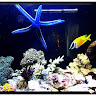# Machine Learning is Fun – Part 7 – What is Regression?

Share
Viewer Rating

Before starting today’s topic, I want to mention a very important point. Please make sure you have covered the concept of the points which I have mentioned in my last post on EDA process.

If you are good with that, then let’s proceed.

So, what is regression? It means trying to find out any relation between or among variables.

Well, so what kind of relation? Linear? Quadratic, or any different curve?

X = Age

Y= Height

Our goal is to find out the relation between age and height. We have some dataset having two columns X and Y. And we are trying to find out the value of Y with the given value of X.

Do you remember the methametical formula in school days?

Y=mX+C

A straight line formula on a 2D plot having X and Y axis.

We are trying to achieve the best fit line if we plot the given data set in a X-Y 2D plane.

As per our experience, the age and height have linear relationship like a straight line.Here is the concept of minimum error.

The concept is we are trying find out a line which have minimum distance between actual Y vs predicted Y in our model.

Looks good right?!

So I request you to google the concept of residual and correlation and OLS method.

OLS means ordinary least square method – which is an algorithm used in linear regression to get the best interception and coefficient value (m and C) to draw the best fit line.

If you put the difference of each Y value with the predicted Y value in a 3D plane of sde( square distance error, m and C), you will come up with a graph like bowl.Now our requirement is to get the minimum point of the bowl like curve, right?

So how to do that?

Simple .. the derivative of each axis (m and C) should be zero for the minimum point of the curve, I mean the point of the end point of the bowl.

By doing this, we shall get the best value of m and C to derive our best fit line.?

I hope you liked this post. I shall discuss on multiple regression on my next post. So stay tuned!IT engineer, Machine Learning enthusiast and aqua hobbyist.